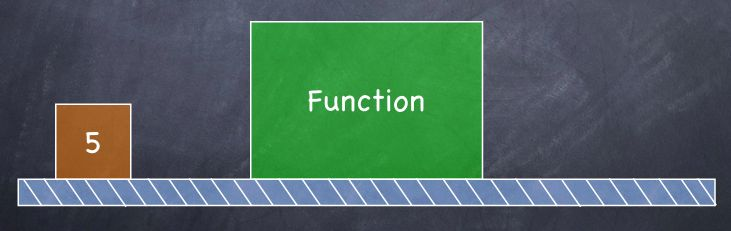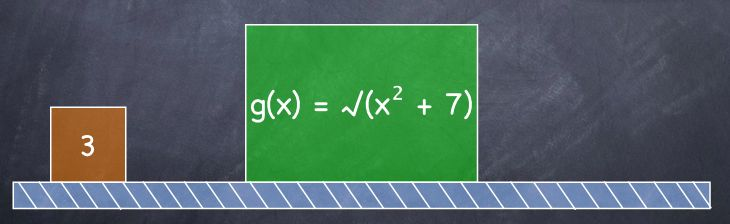Learn the 4 Things You Must Know Before Taking the GMAT!   Download it Now!

# How to Solve Algebraic Functions on the GMAT

Among the GMAT algebra concepts tested on the GMAT are Algebraic Functions. You may remember these from your high school math class as questions that start something like f(x) = …. or g(x) = …. You may also remember having to answer questions involving graphs of functions, like the one you see in Figure 1 (right). Most often, the GMAT tests functions in the form of defined operations questions (you know, those questions involving weird symbols that have no mathematical meaning like or & or — see our lesson on “Common GMAT Word Problems” for more). But occasionally, you’ll see straight-up GMAT functions, so let’s make sure you know how to handle them.

### What is a function?

Let me start by re-phrasing the question of what is a function. It’s not so much what a function is, but rather what a function does. In fact, here’s a useful definition of a function:

Definition: A function assigns to each number in its domain another number.

Looks pretty simple, doesn’t it? It is. Let’s take a closer look at exactly what this means. Really, it means that a function does exactly what the definition says it does. For example, I might have an algebraic function that takes the number 2 (we’ll call it the input) and assigns to it the number 4 (the output). Literally. After the function performs its action on the number 2, it turns into the number 4. Pretty crazy, huh? Likewise, the same algebraic function might take the input number 3 and turn it into the output number 9. And it might take the number 4 and turn it into 16. And so on.

Notation-wise, we’ll use the letter f to represent the function, and so the assignments I’ve just described can be written this way:

f(2) = 4
f(3) = 9
f(4) = 16

Now, what do you notice about these assignments? Do you recognize a pattern? Certainly. In lay terms, what the function is doing is that it’s taking the input, squaring it, and producing the output. As such, rather than having to write out every single possible input and output combination to represent the function, we can simply write a generic function that describes this rule:

f(x) = x²

That way, we can take any input we want, represented here by the variable x, and then do what the function tells us to do to it — namely, to square it — to get the corresponding output. Those values can then be plotted on a graph, by the way, which is actually what you see in Figure 1. Every input x has exactly one output f(x). It’s that simple.

One more quick note about the definition of a function. Notice that it says a function assigns to each number in its domain another number. A domain is simply the allowable input values for the particular function. For example, consider this function:

f(x) = (x+1) / x

What is the domain of this function? Answer: Every single real number except for 0, because remember that you can’t divide by 0. Truthfully, this is a nuance to the definition that you really don’t need to know on the GMAT, but I thought I’d at least mention it since you may have been curious about that part of the definition.

### Understanding Functions: A Useful Analogy

You might find it helpful to think of functions in terms of an assembly line at a factory. There’s some sort of input (in the figure below, the number 5) that’s moving along the conveyor belt and enters the “function” like this:The function will have a very specific rule that tells our hypothetical “machine” what to do with the input. In this example (below), the function tells the machine to take the input (x), square it, and then add the result to the original input x. That’s the function’s rule explained in plain English, but that’s really all a function is to begin with.You see that after the input (5 in our example) “enters” the function, the resulting output is 30.That’s because 5² + 5 = 30. So, if f(x) = x² + x, then f(5) = 30. Make sense?

### Combining Functions

Once you understand how to solve basic functions, like in the example above, you can solve more difficult “combined functions” questions. Just think of the assembly line analogy as we look at this example:

If f(x) = √x and g(x) = √(x² + 7), then what is the value of f(g(3))?

(A) 1
(B) 2
(C) 3
(D) 4
(E) 5

This is like a function inside of a function, isn’t it? In other words, g(3) is the entire input of the function f, right? Thinking back to our assembly line analogy, it would look like this:

So, obviously, we can’t solve this function until we first solve g(3). Once we do, we’ll have the input to plug in to the function f. So, let’s solve g(3) first. The rule for the function g is that g(x) = √(x² + 7), so it’ll look like this:Solving that is pretty easy, right? You simply plug in 3 for x and get √(3² + 7) = √16 = 4. That’s what g(3) equals.

Four (4), then, becomes the new input for the function f:Since the rule for function f is that f(x) = √x, then f(4) = √4 = 2. The correct answer is B. Nice job if you got that!

### Functions on the GMAT

Now that you know the basics of algebraic functions and have seen several sample GMAT problem solving questions in action, try your hand at this more difficult GMAT functions question that will bring it all together for you:

Question: For which of the following functions is f(a + b) = f(a) + f(b) for all positive numbers a and b?

(A) f(x) = x²
(B) f(x) = x + 1
(C) f(x) = √x
(D) f(x) = 2/x
(E) f(x) = -3x

For a detailed answer explanation, watch this video:

Use this newfound GMAT algebra knowledge to go out and dominate the GMAT!